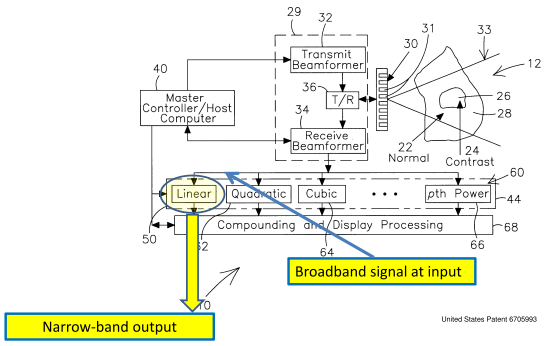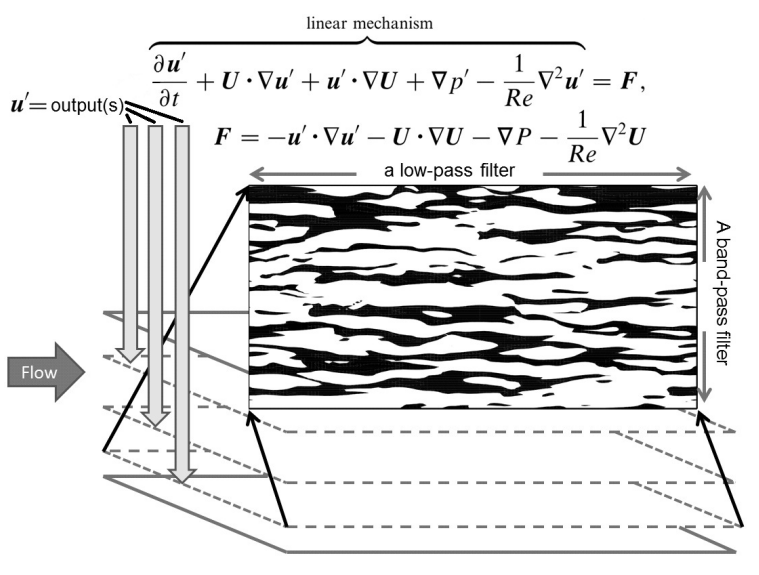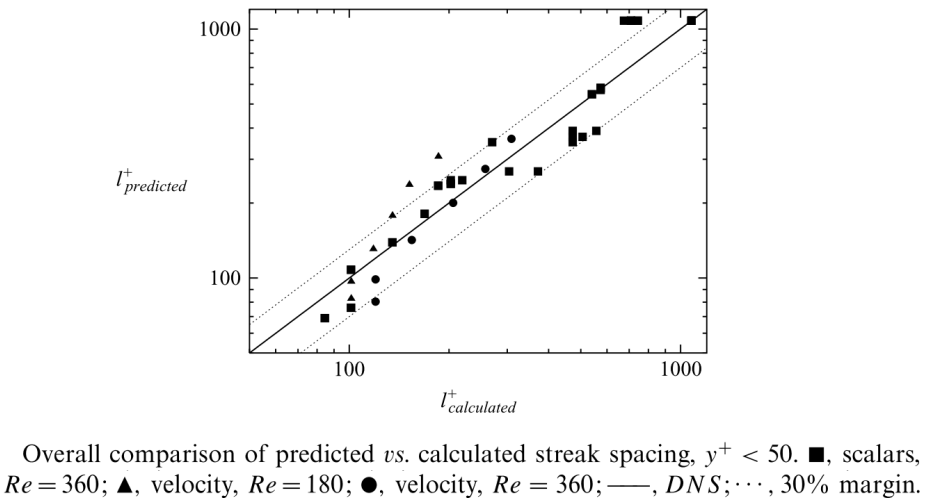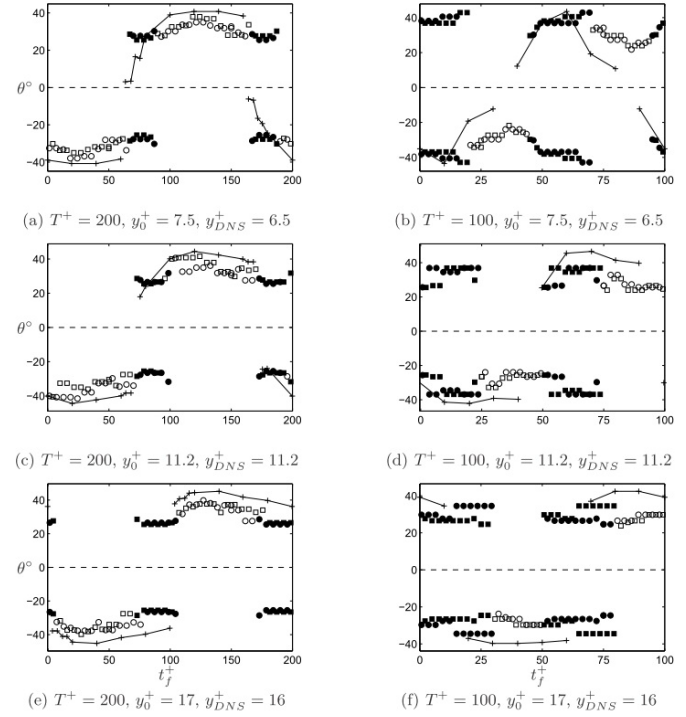# Applying linearised Navier-Stokes equations to developed turbulence: organised structures

Turbulence is dominated by non-linear interactions. Then, how is it possible that the analysis based on linearised equations can lead to a valid prediction about a turbulent flow? One can easily jump to the conclusion that in cases when a linearised approach works the non-linearity has to be in some sense small. This is indeed the case in certain flows. However, this is not the case in near-wall flows where streaks are formed. In such flows the non-linear terms are not small. In the case of streak formation the applicability of linearised equations has a different nature.Fig.1. A non-linear system with a broadband signal at the input to a linear narrow-pass filer.

Let us first consider a simple example. Figure 1 shows a non-linear device containing a linear filter and a number of non-linear elements. Suppose that in this system there are broadband fluctuations. Suppose also that the filter is a narrow-pass filter. Then, while at the input of the filter the signal is broadband, the output of the filter will be a narrow-band signal: it will have a dominant frequency. In a nutshell, this is the mechanism of formation of streaks in near-wall turbulent flows due to selective amplification, or filtering, effect. Other structures might also be formed similarly.

One can disassemble the device, take the filter into the laboratory, and study it there, separately. As a result of this linear analysis (the filter itself is linear, and no non-linear components were brought into the laboratory) one can determine the band-pass properties and, hence, will be able to predict the dominant frequency at the output of the filter when it works as a part of the non-linear system. For this only a linear analysis is required, and the non-linear effects in the full system need not be small. If the goal is to determine the middle of the pass-band, one can solve the optimisation problem consisting in maximising the filter gain over the frequency. This simple idea, transferred to the case of the Navier-Stokes equations, leads to the use of optimal perturbation approaches for predicting patterns in developed turbulent flows.

Transferring the idea of filtering to the Navier-Stokes equations implies partitioning these equations into a linear-filter part and the rest:Fig.2. Visualisation planes at different distance to the wall are possible selections of the filter output.

Experience showed that selecting the base flow U to coincide with the mean velocity profile gives best results in predicting streaks. Then, to find the preferred patterns one can maximise a measure of the solution of the linearised Navier-Stokes equations (the output) over all possible forcing (the input F ) of a ﬁxed amplitude. The output itself is a three-dimensional time-dependent velocity field. In predicting streaks one should restrict it to the visualisation plane, for which the prediction is to be done. One can think about this as a situation when the filter has several outputs, as illustrated by Figure 2. As a measure of the output one can use the energy norm of the velocity field in the visualisation plane. In contrast, the magnitude of the input has to be measure over a large volume. In the context of optimal perturbation approach the situation when the input and the output are measured with different norms can be called a case of a generalised optimal perturbation.

In reality, in turbulent flows the "broadband noise" F is not fully unstructured, and therefore affects the structure of the output. At the same time, the "narrow-pass" filter described by the linearised Navier-Stokes equations has a not-so-narrow band. Experience shows that in the case of near-wall streaks the filtering effect is dominant, but the predicted spacing can deviate from the observation by a factor of up to 2, even though it is more typical to stay within 30%.

The following two figures give an idea of the typical accuracy that can be obtained for streak spacing predicted on the basis of the filtering idea.Fig.3.

Figure 3 is for streak spacing in a flow with a passive scalar admixture. The streaks of both velocity and the passive scalar are observed in direct numerical simulation and predicted using filtering idea. Variation in streak spacing is due to the variation of the distance from the wall to the visualisation plane, the Reynolds number, the nature of the streaks (velocity or passive scalar), and the shape of the mean profile of the passive scalar, which strongly affects the streak spacing. The predicted values are plotted versus the calculated values, so that the exact agreement corresponds to the diagonal shown with a solid line. Dotted lines are 30% error margins. Note the logarithmic scale: this is a comparison over an about of order-of-magnitude variation of the predicted and calculated quantity.Fig.4. Streak angle as a function of time for different values of the oscillation period and for different values of the distance to the oscillating wall.

Figure 4 shows the comparison of the angle of the oblique streaks observed in the flow past an oscillating wall in the regime with turbulent drag reduction. In such a flow the streak angle varies with time. Note that in this case the error in streak spacing prediction was as large as a factor of 2.

Overall, Figures 3 and 4 give a fair representation of the predictive ability and limitations of the approach based on the idea of filtering. It should be stressed that restricting the measure of the magnitude of the output to the magnitude of the output in the visualisation plane for which the comparison is to be made is crucial in making quantitative predictions.